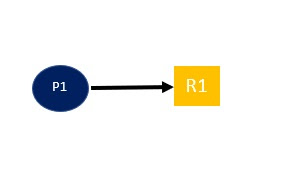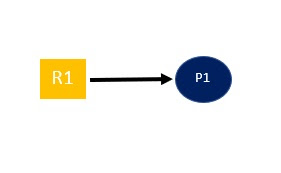Job Saarnee

# Introduction to Dead lock in Operating System

A dead lock is situation in which two or more process
waiting on same event to happen which is never going to be happen then we say those

In order to analyze Dead lock in any system we make use of Resource allocation graph.

# Resource allocation graph

Resource allocation
graph is a graph contain vertices and Edges which represent the Resource,
allocation and Request status for each and every process.

Resource allocation
graph = G (V, E) where V is Process or Resources and E is allocation or
Request.

In above resource
allocation graph P1 does not leave the resource R1 and cannot stop requesting
for R2 similarly P2 does not leave the resource R2 and cannot stop requesting
for R1 they are trying to execute thus there is dead lock.

In above case it is
seem like dead lock but actually it is not dead lock. Because process P3 and P4
require only one resources which is already allotted thus they execute and
release the resources.

Notation used to represent Resource allocation graph.

Process will be
represented by circle

Resources will be
represented by Rectangle

A Resource can have
Single instance or Multiple instance

Here is example of
single instance

Request is
represented as followingP1 is requesting for one  instance of R1

Allocation is
represented as followingOne instance of R1 is  allocated to P1

Q1 Consider a system with n Process and 6 tape drive
and if each process requires two tap drive to complete their execution, then
what is maximum value of n which ensure dead lock?

The solution is quite easy, let first we check what is given in the question

We have n process and 6 tape drive

each process require two tapes

so we first allocate 1 instance of tape drive to each process

P1 – 1

P2 – 1

P3 – 1

P4 – 1

P5 – 1

P6 – 1

as we have only 6 instance of resources, we need one more resources to satisfies the need of each process thus we reduce one process from the above scenario. that’s mean we can able to execute 5 maximum process without any deadlock.

Q2: Consider a system with 3 process each require 2 unit of resources R to complete the execution then what is the minimum number of resources are required to ensure dead lock ?

Solution:

As it is given in the question we have 3 process and each require 2 unit of resource thus first we allocate one instance to each process like
P1- 1
P2- 1
P3- 1
now we need one more resource to satisfy  need of any process after completion that process release 2 resources which is sufficient to satisfy need of any remaining process.
Q3 Consider a system with n Process and 6 tape drive and if each process requires three tap drive to complete their execution, then what is maximum value of n which ensure dead lock?

Solution

This question is little tricky

here we are not going to assign one resources one by one instead of this we take 2 resources at a time like

P1 – 2

P2 – 2

P3 – 2

but this will not ensure deadlock free execution, as there one scenario in which there three process can execute without creating any deadlock like

P1 – 3

P2 – 2

P3 – 1

but as it is not fully ensuring dead lock so 3 is not a right answer

thus right answer is 2 processes, means 2 is the maximum number process which can executed in this scenario without creating deadlock.

Q4: Consider a system with P1, P2, P3 process each require 5, 9, 13 unit of resources R respectively to complete the execution then what is the minimum number of resources are required to ensure dead lock?

Solution:

In question there is need of little bit understanding that first we assign one recourse less then its need like

P1 – 4

P2 – 9

P3 – 12

now system needs one more instance of resource R to complete the need of any one process, than after executing any one processes we get plenty amount of resources to satisfy the need of other process.

here is list of related post